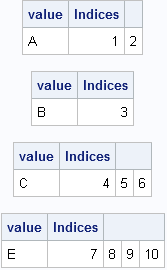I want to create a vector as follows. Suppose there are two given vectors x=[A B C] and f=[1 2 3]. Here f indicates the frequency vector. I hope to generate a vector c=[A B B C C C]. I am trying to use the REPEAT function in the SAS/IML, language but there is always something wrong. Can you help me?

This is probably a good time to remind everyone about the SAS/IML Community (formerly known as a Discussion Forum). You can post your SAS/IML questions there 24 hours a day. That is always a better plan than making a personal appeal to me, because I receive dozens of questions like this every month, and there is no way that I can personally reply. There are lots of experienced SAS/IML experts out there, so please use the SAS/IML Community to tap into that knowledge.

That said, I think the answer to this reader's question makes an interesting example of statistical programming with SAS/IML software. It is trivial to solve this in the DATA step (see the end of this article), but how might you solve it in the SAS/IML language? If you'd like to try to solve this problem yourself, stop reading here. Spoilers ahead!

### Create a new vector that duplicates frequencies

The goal is to write a function that duplicates or "expands" data that have a frequency variable. The important function to use for this task is the CUSUM function, which computes the cumulative frequencies. Let's look at a simple example and apply the CUSUM function to the frequency vector:

```proc iml; values={A,B,C,E}; freq = {2,1,3,4}; cumfreq = cusum(freq); print values freq cumfreq;```As shown in the output, the cumfreq variable contains the indices for the expanded data. The expanded data will be a vector that contains 10 elements. The first data value (A) repeats twice (the freq value), so it repeats until element 2 (the cumfreq value) in the expanded vector. The second category fills element 3. The next category repeats 3 times, so it occupies up through element 6 in the expanded vector. The last category repeats until element 10. The following DO loop specifies each data value and the indices of the expanded vector that it should occupy:

```print (values)[label="value"] (1:cumFreq)[label="Indices"]; do i = 2 to nrow(values); bIdx = 1 + cumFreq[i-1]; /* begin index */ eIdx = cumFreq[i]; /* end index */ value = values[i]; print value (bIdx:eIdx)[label="Indices"]; end;```The output shows that we have all the information we need to allocate a vector of length 10 and fill it with the data values, where the ith value is repeated freq[i] times. The key, it turns out, is to use the CUSUM function to find the indices that correspond to the each data value.

### A module to compute the expanded data

In SAS procedures that support a FREQ statement, the frequency values must be positive integers. If the frequency value is missing or is a nonpositive value, the corresponding data value is excluded from the analysis. It is easy to add that same feature to a module that takes a vector of values and a vector of frequencies and returns a vector that contains the data in expanded form. This is implemented in the following SAS/IML module, which allocates the result vector with the first data value in order to avoid handling the first element outside of the DO loop:

```start expandFreq(_x, _freq); /* Optional: handle nonpositive and fractional frequencies */ idx = loc(_freq > 0); /* trick: in SAS this also handles missing alues */ if ncol(idx)=0 then return (.); x = _x[idx]; freq = round( _freq[idx] );   /* all frequencies are now positive integers */ cumfreq = cusum(freq);   /* Initialize result with x to get correct char/num type */ N = nrow(x); expand = j(cumfreq[N], 1, x); /* useful trick */   do i = 2 to N; bIdx = 1 + cumFreq[i-1]; /* begin index */ eIdx = cumFreq[i]; /* end index */ expand[bIdx:eIdx] = x[i];/* you could use the REPEAT function here */ end; return ( expand ); finish;   /* test the module */ values={A,B,C,D,E,F}; freq = {2,1,3,0,4,.}; /* include nonpositive and missing frequencies */ y = expandFreq(values, freq); print values freq y;```Notice that you don't actually need to use the REPEAT function because SAS/IML is happy to assign a scalar value into a vector. The scalar is automatically repeated as often as needed in order to fill the vector.

### A DATA step solution

As indicated at the beginning of this post, the DATA step solution is quite simple: merely use the OUTPUT statement in a loop, as shown in the following example:

```data Orig; input x \$ Freq; datalines; A 2 B 1 C 3 D 0 E 4 F . ; run;   /* expand original data by frequency variable */ data Expand; keep x; set Orig; if Freq<1 then delete; do i = 1 to int(Freq); output; end; run; proc print data=Expand; run;```

The output data set contains the same data as the y vector in the SAS/IML program.